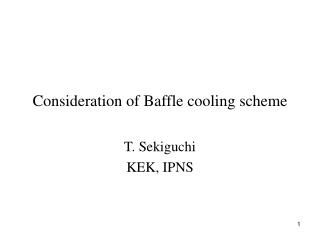Download PresentationConsideration of Baffle cooling scheme

# Consideration of Baffle cooling scheme

Download Presentation## Consideration of Baffle cooling scheme

- - - - - - - - - - - - - - - - - - - - - - - - - - - E N D - - - - - - - - - - - - - - - - - - - - - - - - - - -
##### Presentation Transcript

1. Consideration of Baffle cooling scheme T. Sekiguchi KEK, IPNS

2. Introduction • This document describes general considerations about cooling schemes. • Baffle = Collimator for 1st Horn • A graphite cylinder:fin= 32mm andfout = 400mm length = 1.7m • Heat load: 4.2 kJ/pulse (1.2 kW) due to beam halo and ~1kW from back-scattered pions. g~2kW heat load is expected. gCooling is required. • For calculations, it is assumed that the heat generation is concentrated at the inner surface. 400mm 32mm 1700mm

3. Consideration of cooling scheme Cooled by Helium or water? Merits Demerits

4. Helium flow 10mmfpipe Option #1 (He cooling) • Heat load concentrates on inner part of Baffle gCooling inner surface is efficient. • Helium is transferred to inner cavity with10mmfpipe. • Open circuit • Hand calculation is performed. • Helium initial temperature = 25 ºC • Very high temperature gas blows toward the beam window and the target! g Closed circuit is preferable.

5. He in He out Option #2 (He cooling) • Closed circuit. • Helium flow is divided into 6 paths. • 6 holes atfhole=200mm fNot optimized. • 2kW heat flow into 6 holes. g heat flow for each hole = 333W. • Helium temperature rise is reduced since only 600W heat is cooled. • Calculation with realistic model is needed. 10mmf 200mm

6. Water flow Option #3 (Water cooling) • Cooling outer surface. • 10 pipes attached on outer surface f Not optimized • 2kW heat flow into 10 pipes. g Heat flow for each pipe = 200W • Heat transfer coeff. is ~ 950W/m2/K with even 1l/min water flow. • Calculations with realistic model is needed. • To reduce the amount of radioactive waste water, the number of pipes and the pipe diameter should be reduced. 10mmfSUS pipe

7. Summary • Helium and water cooling are considered. • Calculations with some heat flow assumptions are performed. • In the case of He cooling, closed circuit is preferable since high temperature gas blows the beam window. • We need FEM calculations with • helium or water flows and • expected thermal load distribution. • Cooling scheme should be optimized in the cases of the options #2 and #3.

8. Supplements

9. Table #1 for calculation Helium properties under 0.1MPa condition.

10. Table #2 for calculation Properties of water.

11. Simple simulations by ANSYS • Rotational symmetry model of the option #2. • Helium flow ~1g/s (0.167g/s per hole), temperature = 410 ºC Heat conductivity around 400 ºC ~80 W/m/K (const.) adiabatic Heat flow at inner surface ~2kW Heat transfer coeff. ~ 121W/m2/K Temperature @inner surface ~440 ºC Hole diameter =10mm

12. Simple simulations by ANSYS • Rotational symmetry model of the option #2. • Helium flow ~1g/s (0.167g/s per hole), temperature = 410 ºC Heat conductivity around 400 ºC ~80 W/m/K (const.) Natural convection ~10W/m2/K Heat flow at inner surface ~2kW Atmospheric temp. ~60 ºC Heat transfer coeff. ~ 121W/m2/K Temperature @inner surface ~360 ºC Hole diameter =10mm

13. Simple simulations by ANSYS • Rotational symmetry model of the option #3. • Water flow ~1l/min (16.7g/s), temperature = 27.9 ºC Heat conductivity around 30 ºC ~116 W/m/K (const.) adiabatic Heat flow at inner surface ~2kW Water pipe d=10mm Heat transfer coeff. ~ 2490 W/m2/K Temperature @ inner surface ~33.7 ºC

14. Simple simulations by ANSYS • Rotational symmetry model of the option #3. • Water flow ~1l/min (16.7g/s), temperature = 27.9 ºC Atmospheric temp ~60 ºC Heat conductivity around 30 ºC ~116 W/m/K (const.) Natural convection ~10W/m2/K Heat flow at inner surface ~2kW Water pipe d=10mm Heat transfer coeff. ~ 2490 W/m2/K Temperature @ inner surface ~34.2 ºC